## Potential of a homogeneous gravitational field

A homogeneous gravitational field is characterized by a constant vector field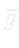, the well known gravitational acceleration. Let us consider some volume or vessel filled with an incompressible fluid of density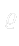. The gravitational field exerts a force on the fluid. Its force density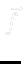is given by the following well known formula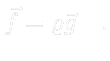It is a simple task to  potential to (). Let us remind, a potential (if it exists) of some given force field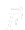is defined as a certain function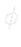satisfying the following equation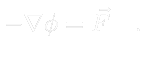We can see that to thegiven by () there exists a potential, let us denote it by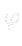, stating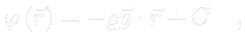where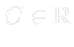is a constant of integration3.3 and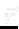is a position vector3.4.